ML Aggarwal Class 7 Solutions Chapter 14 Symmetry Ex 14.2 for ICSE Understanding Mathematics acts as the best resource during your learning and helps you score well in your exams.

## ML Aggarwal Class 7 Solutions for ICSE Maths Chapter 14 Symmetry Ex 14.2

Question 1.
Which of the following figures have rotational symmetry? In the case of rotational symmetry, find the order of rotational symmetry.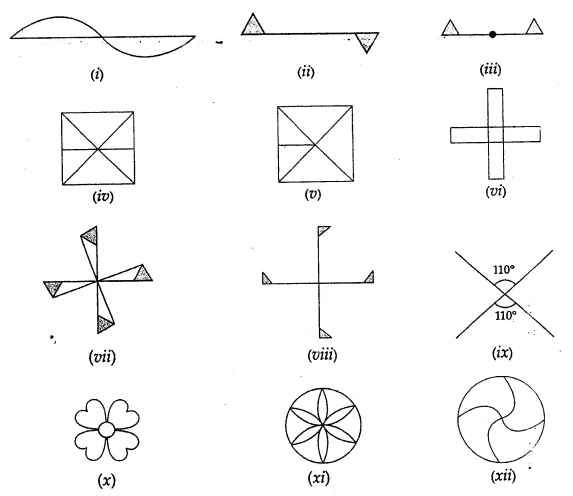Solution:Question 2.
Which of the following figures have rotational symmetry of order greater than 1?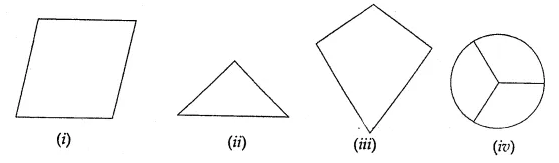Solution: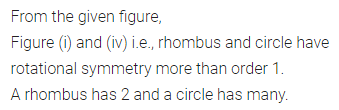Question 3.
Name any two figures that have both lines of symmetry and rotational symmetry.
Solution: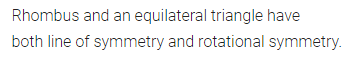Question 4.
Name the quadrilaterals which have both line and rotational symmetry of order more than 1.
Solution: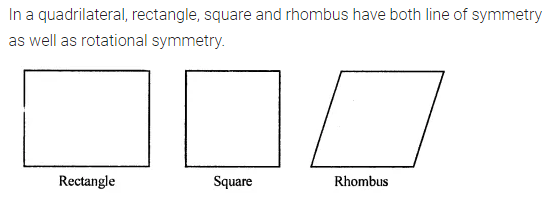Question 5.
Draw a rough sketch of:
(i) a triangle with both line and rotational symmetries of order more than 1.
(ii) a triangle with only one line of symmetry and no rotational symmetry of order more than 1.
(iii) a triangle with no line symmetry but rotational symmetry of order 1.
(iv) a quadrilateral with no line symmetry but rotational symmetry of order more than 1.
(v) a quadrilateral with line symmetry but not rotational symmetry of order more than 1.
Solution: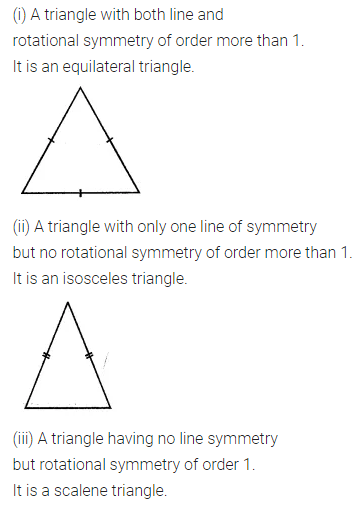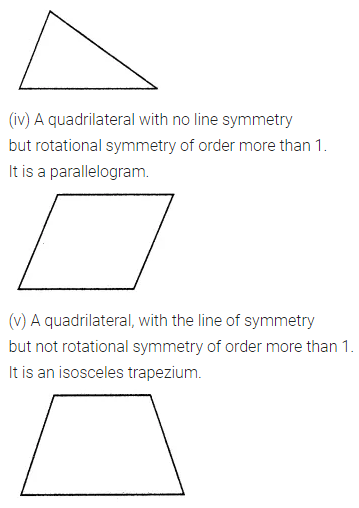Question 6.
If a figure has two or more than two lines of symmetry, can it have rotational symmetry of order more than one?
Solution: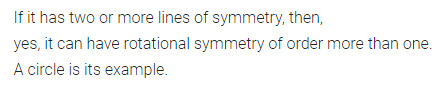Question 7.
A figure looks exactly the same as its original figure after rotation of 60°. At what other angles will this figure appear the same?
What can you say if the angle of rotation is
(i) 72°
(ii) 45°
(iii) 50°?
Solution: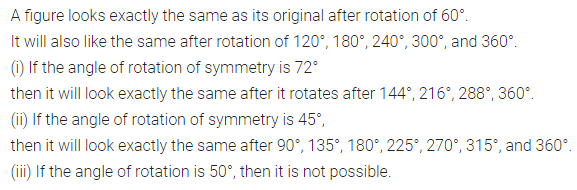Question 8.
Can a figure possessing rotational symmetry have an angle of rotation of measure
(i) 180°
(ii) 120°
(iii) 90°
(iv) 30°
(v) 15°
(vi) 17°?
Solution: